History of Computation - Prehistory

## 2.1. Introduction

Although the computer and its widespread application in our society, are phenomena that have become predominant in only the last 30 years, many of the concepts underlying these developments have their origins in concerns dating back to the earliest cultures. Computers manipulate data (Latin, plural of datum, neut. p.p. dare, cognate Sanskrit, datta: those things which have been given), i.e. process and transform given representations of information in order to obtain a desired result. Within this basic description of computer behaviour we can discern two fundamental ideas:

• Representation: A concrete, symbolic encoding of information, e.g. numbers, words, names.
• Transformation: The steps (recipe, program, algorithm) used to calculate a specific result.
A symbolic encoding of information provides a vehicle for communication - information can be passed on in a commonly understood form. A record of the process by which the representation is transformed allows the calculation process to be carried out repeatedly on different sets of data, e.g. we have all learned the steps needed to determine the result of multiplying any two large numbers.

One might ask, however, why, given a system for encoding information and the sequence of steps needed to manipulate this to a specific end, it should be necessary to seek mechanical assistance with the task? The answer to this question lies in the fact that the calculations required to carry out these tasks are often laborious. This fact has two consequences if no mechanical aid is employed:

• The computation will take a long time to complete.
• The answers may be incorrect, because of human error, and so the same computation may have to be carried out several times.
(Consider, which would you prefer: to multiply two 5 figure numbers by hand on paper or to use an electronic calculator? Which answer would you have greater confidence in?)

The calculations involved in predicting celestial phenomena from previously observed data; in assessing the rates of taxation to levy in order to raise a required sum; in analysing census statistics; in determining the path of a projectile; all of these are examples where lengthy, tedious and (if done by hand) error-prone computations arise.

Thus the historical development of the topic we are considering can be seen to be based on three related processes:

• The development of symbolic representations of information that are amenable to manipulation.
• The formulation of algorithms by which such representations may be processed to solve computational problems.
• The construction of mechanical aids that allow such algorithms to be implemented in an efficient and a reliable manner.
It ought to be clear that methods for representing information must been have developed first, so it is appropriate to examine one aspect of such representations - number systems - before going on to consider the algorithms and mechanical aids that utilise them.

The simplest method of representing numbers is to use a sequence of identical marks to denote quantities, e.g. Figure 1 below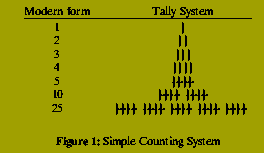Archaeological discoveries have established that the `tally system' was independently developed by many early cultures, e.g. bones with notches denoting quantities have been found by anthropologists in Czechoslovakia, similar fragments dating from around 8500 B.C. have also been discovered in Africa. Despite its rudimentary nature, this system does exhibit important features that were to be preserved in later systems. The most important of these is the concept of counting in multiples of some basic number (5 in the example above). While most societies adopted 5 or 10 as the typical base (from the practice of counting on fingers) these were by no means universally chosen. The South American Mayan culture employed a system based around the number 360 (from their estimate of the number of days in a year); the Babylonians used 60 as a base. It is interesting to note that the influence of the Mayan and Babylonian systems continues in the present day (a circle contains 360 degrees, a degree 60 subparts called minutes, a minute 60 subparts called seconds; similarly we denote time units in multiples of 60 - 1 hour is 60 minutes is 60 times 60 seconds)

Although the tally system has several advantages - it is easy to understand, simple arithmetic operations such as addition, subtraction and multiplication can be performed without great difficulty - it is extremely cumbersome when used to represent large numbers, such as might arise in recording population sizes, and it is not suitable for more complicated computational tasks, e.g. division. Attempts to address the first problem can be discerned in the notational systems used by Greek and Roman societies (from ca. 1000 B.C for Greek, 700 B.C, Roman). These, though, were really only slightly more sophisticated versions of the tally system: instead of using a single denotational symbol to construct numbers, a set of different symbols is employed, each representing a different quantity, Figure 2.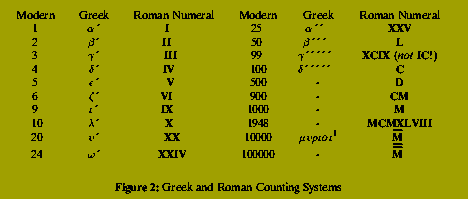1) {muupsilonrhoiotaoiota} is typically translated as {10,000} but is more accurately rendered as `countless'. Herodotus' estimate of the Persian forces at the Battle of Marathon and the source of the English word `myriad'.

Notice that both of these systems eventually become no better (and in some respects considerably worse) than the simple tally system. The method of Roman numerals does, however, introduce an important idea, although failing to exploit it fully: the concept of positional notation. Thus in the representation of 1948 the opening M has a different meaning from the M occurring as the third character. Both of these systems indicate the importance of the qualification `amenable to manipulation' that we stressed earlier. The major deficiency of the Roman system is that it is decidedly unsuitable as a basis for computation. In the tally system the calculation of 494 + 506 is a (notationally) lengthy but very easy computation; the computation of fBCDXCIVfR + fBDVIfR in Roman numerals is not (the answer, M, bears no symbolic relation to the summands and although the result is almost twice as large as the individual contributions, it is expressed using one symbol instead of nine). A task such as multiplication, conceivable in the tally system, present major difficulties in Roman numerals. So inflexible is this system that a suitable topic for doctoral research in the early European universities concerned algorithms for multiplication and division using Roman numerals. Despite all of these drawbacks, Roman numerals remained the predominant means of representing quantities in European culture well into the 14th century.

They were ultimately replaced by a system which contributed what was one of the most important discoveries of early science: a fully positional notation with a representation for the number zero. There is evidence that the Mayan civilisation employed a symbol for zero in their number system. Its arrival in European science came via knowledge of Arabic mathematics. Arab scholars had themselves learned of this system from Indian civilisation. The Indian discovery dates from, at the latest, 200 B.C (the earliest recorded use, in a textbook, by Bakhshali). The word `zero' in English, itself originates from India (Sanskrit sunya - empty or blank - translated as zifr by Arab writers, hence Latin zephirum and English zero and cipher). The Arabic system employed 10 different symbols representing the numbers 0,1,2,...,9 and formed the basis of the decimal system that is used today. First popularised in Europe by Leonardo of Pisa in his Liber Abaci (The Book of Computation) of 1228, despite attempts to suppress it during the reaction against Islamic scholarship, the obvious advantages of the system over Roman numerals eventually led to its being universally adopted.

We now turn to the development of the earliest algorithms and mechanical computing devices.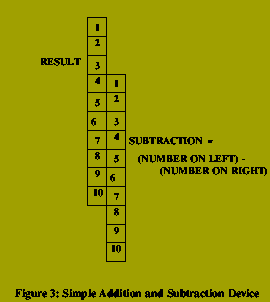Figure 3 shows the structure of one of the earliest forms of primitive computing device that could be used for addition and subtraction. Units were marked out on two lengths of wood. Addition tables and the result of subtractions could be constructed by lining up appropriate units. Other than such basic mechanisms, look-up tables were constructed. At Senkereh in what was Babylonia a clay tablet dating from between 2300-1600 B.C has been discovered which contains the squares of the first 24 numbers. This is now in the British Museum. Such tables obviate the need to recompute particular values and were probably constructed using stones as counters, e.g. Figure 4.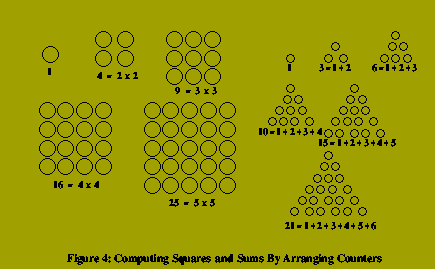The use of pebbles and arrangements of these as aids to computing was common to many cultures. Its influence is apparent in many terms still used today, e.g square (via arrangements in Figure 4), triangular numbers which represent the sums of the first n numbers; calculus and calculation both deriving from the Latin for pebble (hence the different usages of the word calculus in mathematical sciences and medicine); cheque and exchequer which derive from the mediaeval English custom of calculating tax levies by piling stones on a board marked out in black and white squares (the word is combination of Latin ex meaning `from' and Norman French cheque for the checked pattern).

The use of counters as an aid to computation reaches its greatest level of sophistication and power in a device which is still very extensively used today in the Far East: the abacus. Figure 5The abacus represents the state of a calculation by the position of beads strung into columns on wires. The earliest invention of the abacus is now credited to the Babylonians (the word abacus derives from a Phoenician word abak). By the sixth century B.C. they were widely used in Greek society: the historian Herodotus (ca. 484-424 B.C) mentions them in this period and left records of complex calculations performed on them, e.g. the accrued interest on a loan of 766 talents, 1095 drachmae and 5 obols over a period of 1464 days at a rate of one drachma per day for every 5 talents. Other incidences of the use of abaci in late Greek culture are Eutocius of Ascalon's computation of (30133/4)^2; references in the surviving speeches of the orator Demosthenes (385-322 B.C); and the writings of the Cynic philosopher Diogenes (d. ca. 320 B.C). It was in Oriental cultures, principally China and Japan, however, that the abacus reached its highest level of development. The Japanese contributed the concept of dividing the abacus frame horizontally into two zones (the so-called Heaven zone with 2 beads and Earth Zone with 5)^2

2) Although the modern Japanese abacus has reduced the heaven zone to a single bead. With this device calculations of great complexity could be performed at great speed. In 1946, in a meeting between the fastest mechanical calculator operator in the U.S. Army, one Private. T.N. Wood equipped with a contemporary state-of-the-art calculator, was defeated in 4 out 5 speed contests by Kiyoshi Matsuzaki, who used an abacus. This device is still widely used in banking and financial calculations in Japan today.

It should be noted that even such crude devices as the notched sticks of Figure 4, presume some understanding of the algorithmic process determining their use. It is likely that the first employers of simple computational aids were unaware that the reason why they produced the correct answer was because they exploited simple algorithms relating the data operated upon to the results obtained. The Greek mathematician, Euclid (4th Century B.C) is the earliest known identifier of specific algorithms (although it is clear that the calculations, such as those documented by Herodotus on abaci - compute the interest due on amount x at a rate of y per z for each w - presuppose methods stating how to proceed). Euclid's work The Elements is of collection of results, mainly in Geometry, spread over 7 books. It is important, for our purposes, for two reasons:

• It is the earliest known attempt to formalise the concept of algorithm, i.e. to separate `admissible' computational processes from `inadmissible' ones.
• Book VII, dedicated to properties of numbers, describes a number of important algorithms at least one of which is still taught today.
As regards the first point, Euclid addressed the issue of what algorithms could be said to be valid if one was concerned with constructing various geometrical objects, e.g. squares with a certain area; lines and angles with particular properties; regular polygons, etc. The Elements admits such an object if and only if one can show how to construct it in a finite number of steps using only a ruler, i.e. straight edge to draw lines, and a pair of compasses (to draw circles and arcs). See Figure 6.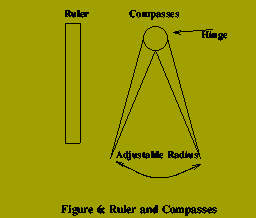For example one may easily check that the algorithm below constructs a regular hexagon - a six sided figure in which all sides have equal length and all internal angles are equal:

• Draw a circle C of radius r (uses compasses)
• Choose a point phi on C and draw a circle with centre phi and radius r. The new circle cuts C at two points alpha and beta.
• Draw a circle with centre alpha and radius r. This cuts C at phi and a new point gamma.
• Draw a circle with centre gamma and radius r. This cuts C at alpha and a new point delta.
• Draw a circle with centre delta and radius r. This cuts C at gamma and a new point epsilon.
• Draw a circle with centre epsilon. This cuts C at delta and beta.
• Use a straight line to connect phi (-> alpha (-> gamma (-> delta (-> epsilon (-> beta (-> phi The resulting polygon is a regular hexagon.

### Ruler and Compass Construction of Regular Hexagon

Stages of this process are illustrated in Figure 7.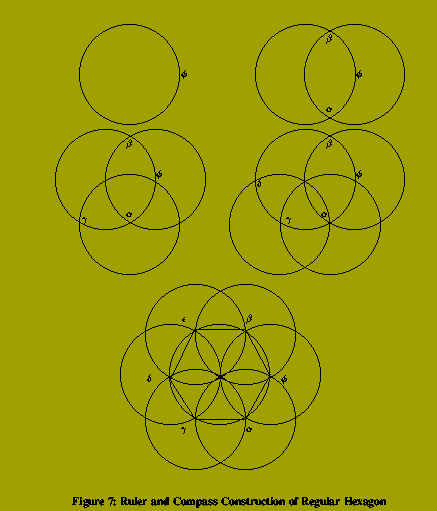Although this may seem a very primitive class of algorithm, problems of great subtlety arise in it. In particular, the problem of squaring the circle (i.e. construct a square whose area is equal to that of a given circle) was first raised by Euclid. This was not shown to be impossible using ruler and compasses until the end of the 19th Century^3 - over 2300 years after Euclid's death.

3) By Lindemann in 1882. Of the other important constructions left open by Euclid - trisection of a given angle and duplication of a given cube - the former was proved impossible by Descartes (1637), the latter was known to be impossible by Arabic mathematicians.

The second important contribution of The Elements is the algorithm to calculate the greatest common divisor of two numbers. Given two numbers - m and n - the greatest common divisor of m and n, denoted gcd( m,n ), is the largest number that divides both m and n without leaving any remainder, e.g. gcd ( 12, 40 ) = 4, gcd ( 7, 15 ) = 1, etc. Euclid's Algorithm, as it is now known, is shown below:

```greatest common divisor of m and n

1. If m is smaller than n then swop the values of m and n.
2. Set m equal to the remainder of m divided by n.
3. If m is not equal to 0 then go back to step 1, with the
new values of n and m.
4. Return n as the answer.
```

Euclid's Algorithm

Euclid's work was not the only early investigation of algorithms. Another important Greek contribution concerns a problem which is still very much of interest today - the generation of prime numbers. A prime number is a whole number, greater than 1, which is divisible without remainder by only itself and by 1. Methods of finding prime numbers have existed for over 2000 years and, since the only known algorithms are computationally very demanding, finding large primes is a standard performance test carried out on new powerful computer systems: in the last 40 years the size of the largest known prime has advanced from a 40 digit number (found in 1886) to a 15,000 digit number (identified in 1991). The first algorithm to identify prime numbers is the famous Sieve of Eratosthenes (fl. 3rd Century B.C). This works as follows: suppose on wishes to know all the prime numbers less than some number, n say. Write down all the numbers from 2 to n and then carry out the following steps:

```1. Let k := 2
2. For each number, m, between k+1 and n if m is an exact
multiple of k then cross m of the list of numbers.
3. Set k to be the smallest uncrossed off number left.
4. If k is less than n then repeat process from step (2).
5. Any number which has not been crossed off is a prime number.
```

### The Sieve of Eratosthenes

The process is illustrated below: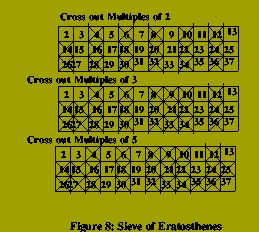The word algorithm in English comes from the Arab mathematician al-Khowarizmi who flourished at the end of the 8th Century A.D. Al-Khowarizmi had reported the Indian discovery of the decimal number system with zero and introduced a number of important concepts in his book Al-jabr wa'l muqabala whose title gives us the word algebra in English. Another Arab mathematician, al-Khashi (1393-1449), devised a method of computing the decimal expansion of pi (the ratio between a circle's circumference and its diameter)^4, obtaining a result accurate to 16 places.

4) A method of memorising this is the phrase: `How I need a drink, alcoholic of course, after all those lectures involving tedious mnemonics for pi'. The number of letters in each word gives the sequence of digits in the expansion of pi.

Al-Khashi also devised the first mechanical computing devices which could be used to predict the occurrence of important celestial phenomena such as solar and lunar eclipses.

In summary, by the middle of the 15th Century there had evolved a flexible and powerful system for representing numerical quantities (both whole numbers and decimal fractions); some basic algorithmic techniques had been developed for manipulating these representations to deal with various calculations; and the first moderately sophisticated aids to such calculations had been developed.

One of the important trends in the history of this topic becomes apparent around this period: that as the level of scientific and engineering knowledge increases, so in parallel does the complexity of the mathematical calculations associated with their development. Thus, to date, there has never been a point reached where the contemporary state of computational aids has exceeded the most demanding requirements of contemporary sciences. We can cite two examples of this at the end of the 15th century. First, increasing knowledge about matters such as planetary motion had resulted in data from observations that was increasingly difficult to process and interpret with the methods available. Second, there was little in the way of devices to help with navigation at a time when there was a considerable increase in exploration and commercial trade. In the next section of these notes we examine the continuing development of computational mechanisms following this period.PED Home Page